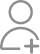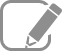Take Class 8 Tuition from the Best Tutors

•Affordable fees
•1-1 or Group class
•Flexible Timings
•Verified Tutors

Search in

# Water is pouring into a cubiodal reservoir at the rate of 60 litres per minute. If the volume of reservoir is 108 m3, find the number of hours it will take to fill the reservoir.

Learn Exercise 11.4Follow 1AnswerVolume of cuboidal reservoir = 108 m3 = (108 × 1000) L = 108000 L It is given that water is being poured at the rate of 60 L per minute. That is, (60 × 60) L = 3600 L per hour Required number of hours = 30 hours Thus, it will take 30 hours to fill the reservoir.

Volume of cuboidal reservoir = 108 m3 = (108 × 1000) L = 108000 L

It is given that water is being poured at the rate of 60 L per minute.

That is, (60 × 60) L = 3600 L per hour

Required number of hours= 30 hours

Thus, it will take 30 hours to fill the reservoir.

Now ask question in any of the 1000+ Categories, and get Answers from Tutors and Trainers on UrbanPro.com

Find Class VIII near you

Looking for Class 8 Tuition ?

Learn from the Best Tutors on UrbanPro

Are you a Tutor or Training Institute?

Join UrbanPro Today to find students near you
X

### Looking for Class 8 Tuition Classes?

The best tutors for Class 8 Tuition Classes are on UrbanPro

• Select the best Tutor
• Book & Attend a Free Demo
• Pay and start Learning### Take Class 8 Tuition with the Best Tutors

The best Tutors for Class 8 Tuition Classes are on UrbanPro